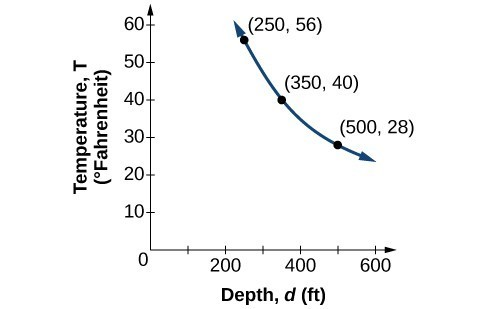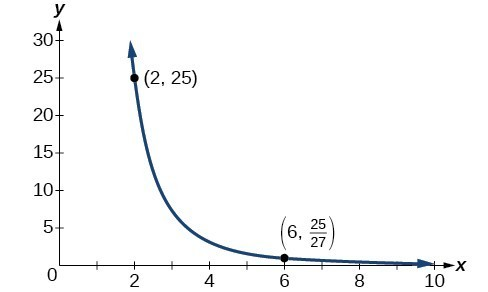## Solve inverse variation problems

Water temperature in an ocean varies inversely to the water’s depth. Between the depths of 250 feet and 500 feet, the formula $T=\frac{14,000}{d}$ gives us the temperature in degrees Fahrenheit at a depth in feet below Earth’s surface. Consider the Atlantic Ocean, which covers 22% of Earth’s surface. At a certain location, at the depth of 500 feet, the temperature may be 28°F.

If we create a table we observe that, as the depth increases, the water temperature decreases.

d, depth $T=\frac{\text{14,000}}{d}$ Interpretation
500 ft $\frac{14,000}{500}=28$ At a depth of 500 ft, the water temperature is 28° F.
350 ft $\frac{14,000}{350}=40$ At a depth of 350 ft, the water temperature is 40° F.
250 ft $\frac{14,000}{250}=56$ At a depth of 250 ft, the water temperature is 56° F.

We notice in the relationship between these variables that, as one quantity increases, the other decreases. The two quantities are said to be inversely proportional and each term varies inversely with the other. Inversely proportional relationships are also called inverse variations.

For our example, the graph depicts the inverse variation. We say the water temperature varies inversely with the depth of the water because, as the depth increases, the temperature decreases. The formula $y=\frac{k}{x}$ for inverse variation in this case uses = 14,000.Figure 3

### A General Note: Inverse Variation

If x and y are related by an equation of the form

$y=\frac{k}{{x}^{n}}$

where k is a nonzero constant, then we say that y varies inversely with the nth power of x. In inversely proportional relationships, or inverse variations, there is a constant multiple $k={x}^{n}y$.

### Example 2: Writing a Formula for an Inversely Proportional Relationship

A tourist plans to drive 100 miles. Find a formula for the time the trip will take as a function of the speed the tourist drives.

### Solution

Recall that multiplying speed by time gives distance. If we let t represent the drive time in hours, and v represent the velocity (speed or rate) at which the tourist drives, then vt = distance. Because the distance is fixed at 100 miles, vt = 100. Solving this relationship for the time gives us our function.

$\begin{cases}t\left(v\right)=\frac{100}{v}\hfill \\ \text{ }=100{v}^{-1}\hfill \end{cases}$

We can see that the constant of variation is 100 and, although we can write the relationship using the negative exponent, it is more common to see it written as a fraction.

### How To: Given a description of an indirect variation problem, solve for an unknown.

1. Identify the input, x, and the output, y.
2. Determine the constant of variation. You may need to multiply y by the specified power of x to determine the constant of variation.
3. Use the constant of variation to write an equation for the relationship.
4. Substitute known values into the equation to find the unknown.

### Example 3: Solving an Inverse Variation Problem

A quantity y varies inversely with the cube of x. If = 25 when = 2, find y when x is 6.

### Solution

The general formula for inverse variation with a cube is $y=\frac{k}{{x}^{3}}$. The constant can be found by multiplying y by the cube of x.

$\begin{cases}k={x}^{3}y\hfill \\ \text{ }={2}^{3}\cdot 25\hfill \\ \text{ }=200\hfill \end{cases}$

Now we use the constant to write an equation that represents this relationship.

$\begin{cases}y=\frac{k}{{x}^{3}},k=200\hfill \\ y=\frac{200}{{x}^{3}}\hfill \end{cases}$

Substitute = 6 and solve for y.

$\begin{cases}y=\frac{200}{{6}^{3}}\hfill \\ \text{ }=\frac{25}{27}\hfill \end{cases}$

### Analysis of the Solution

The graph of this equation is a rational function.Figure 4

### Try It 2

A quantity y varies inversely with the square of x. If = 8 when = 3, find y when x is 4.

Solution